Click to Chat

1800-1023-196

+91-120-4616500

CART 0

• 0

MY CART (5)

Use Coupon: CART20 and get 20% off on all online Study Material

ITEM
DETAILS
MRP
DISCOUNT
FINAL PRICE
Total Price: Rs.

There are no items in this cart.
Continue Shopping• Complete Physics Course - Class 11
• OFFERED PRICE: Rs. 2,968
• View Details

Surface Tension

Table of Content

How do Detergents Clean Dirty Clothes?

Properties of Surface Tension

Surface Energy

Simulation for Size of Bubble as a Comparison With Depth

Force Approach

Angle of Contact

Case I: When q < 90o

Case II: When q > 90o

Capillarity

Rise of Liquid in a tube of Insufficient Length

Energy Required to Raise a Liquid in a Capillary tube

Stokes' Law and Terminal Velocity

Related Resources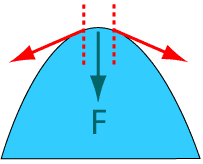“Surface tension is the property of a liquid by virtue of which its free surface behaves like a stretched membrane and supports, comparatively heavier objects placed over it”. It is measured in terms of force of surface tension.

The free surface of a liquid contracts so that its exposed surface area is a minimum, i.e., it behaves as if it were under tension, somewhat like a stretched elastic membrane. This property is known as surface tension. The surface tension of a liquid varies with temperature as well as dissolved impurities, etc. When soap is mixed with water, the surface tension of water decreases. Also, the surface tension decreases with increase in temperature.

How do Detergents Clean Dirty Clothes?

Consider a wire frame (see the adjacent figure) equipped with a sliding wire AB. It is dipped in a soapy water. A film of liquid is formed on it. A force F has to be applied to hold the wire in place. Since the soap film has two surfaces attached to the wire, the total length of the film in contact with the wire is 2L.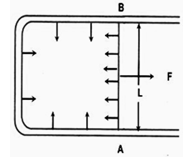T (surface tension) = F/2L.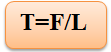Surface tension of a liquid is measured by the normal force acting per unit length. On either side of an imaginary line drawn on the free surface of a liquid, the direction of this force is perpendicular to the line and tangential to the free surface of liquid.

Properties of Surface Tension

Scalar quantity.

Temperature sensitive.

Impurity sensitive.

Depends only n the nature of the liquid.

Unit of surface tension, N/m.

Dimension of surface tension, ML0T-2.

Surface Energy

If the area of the liquid surface has to be increased, work has to be done against the force of surface tension. The work done to from a film is stored as potential energy in the surface and the amount of this energy per unit area of this surface under isothermal condition is the "intrinsic surface energy" or free surface energy density.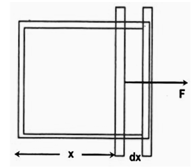Work done in small displacement dx

dW = F × dx = 2TL dx

= 2TLx = TA

As A = 2Lx (area of both sides),

W/A = T (intrinsic surface energy)

Question:-

What is the surface energy of a soap bubble of radius r?

E = TA = T × 4Πr2 × 2 (as it has two surfaces)

= 8Πr2 T.

_____________________________________________________________________________________________

Question:-

What is the surface energy of an air bubble inside a soap solution?

E = T × A = 4Πr2T, as it has only one surface

Excess Pressure

The pressure inside a soap bubble and that outside it are not identical due to surface tension of the soap bubble. To calculate this pressure difference, let's first consider an air bubble inside a liquid. If the pressure difference is Δp, then the work done to increase the radius of the bubble from r to
(r + Δr) is given by:

W = FΔr = 4Πr2 Δp Δr

Change in area

ΔS = 4Π (r + Δr)2 - 4Πr2 = 8ΠrΔr.

From the definition of surface tension

T = W/ΔS = (4Πr2 Δp Δr)/(8ΠrΔr) or Δp = 2T/r

For a soap bubble in air, there are two surfaces.

So, Δp = 2 × 2T/r = 4T/r

Simulation for Size of Bubble as a Comparison With Depth

Bubble bubble boil and trouble. This animaition is used to show size as a comparison with depth. The user move the bubble around while making observations of size versus depth. Then the student can make inferences as to why they get this behavior.

Force Approach

Consider the equilibrium of a hemispherical portion of a liquid bubble of radius R and surface tension T as shown in the figure. For the equilibrium of the liquid bubble.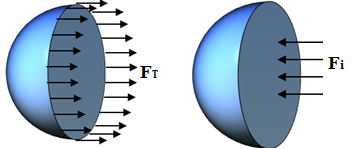F0 - Fi + FT = 0 = 0 where F0 = force due to the outside pressure.

Fi = force due to the inside pressure

FT = force due to the surface tension

P0ΠR2 - PiΠR2 + 2(2ΠRT) = 0

=> Pi - P0 = 4T/R

where R is the radius of the bubble.

Two soap bubbles of different radii (r, R: r < R) are connected by means of a tube. What will happen to the larger bubble?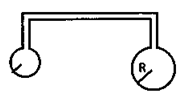Angle of Contact

1. Angle of contact, for a solid and a liquid, is defined as the angle between tangent to the liquid surface drawn at the point of contact and the solid surface inside the liquid.2. The angle of contact of a liquid surface on a solid surface depends on the nature of the liquid and the solid.

Case I: When q < 90o

The liquid surface curves up towards the solid. This happens when the force of cohesion between two liquid molecules is less than force of adhesion between the liquid and the solid. If such a liquid is poured into a solid tube, it will have a concave meniscus. For example, a glass rod dipped in water or water inside a glass tube.

Case II: When q > 90o

The liquid surfaces get curved downward in contact with a solid. In this case, the force of cohesion is greater than the force of adhesion. In such cases, solids do not get "wet". When such liquids are put into a solid tube, a convex meniscus is obtained.

For example, a glass rod dipped in mercury or mercury within a solid glass tube.

Capillarity

When a piece of chalk is dipped into water, it is observed that water rises through the pores of the chalk and wets it.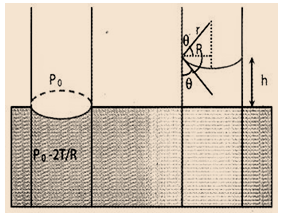Consider a glass capillary of radius R dipped in water as shown in the figure. The pressure below the meniscus will be (p0 - 2T/r). To compensate for this pressure difference, water in the capillary rises so that

2T/r = rgh     or     h = 2T/rgρ

where r in the radius of meniscus,

r = R/cos θ

where q is the angle of contact.

Thus,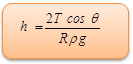If q < 90o, the meniscus will be concave, for illustration: at a water-glass interface.

If q < 90o, the meniscus will be convex, for illustration: at a mercury-glass interface.

Rise of Liquid in a tube of Insufficient Length

We have seen, how a liquid rises up into a capillary tube, dipped into it, until the weight of the liquid in the tube is just balanced by the force due to its surface tension. If q be the angle of contact between the liquid and the tube, and R, the radius of liquid meniscus in the tube, we have r = R cos q, where r is the radius of the tube; so that above relation now becomes,T = (Rcos θ.hρ.g)/(2 cos θ )=(R.h.ρ.g)/2

where h is the height of the liquid column in the tube

Here, clearly

R.h = 2T/ρg, a constant

Now with the tube sufficiently longer than h, it is the value of h alone that changes to satisfy the above relation for T. But if the tube be smaller than the calculated value of h, the only variable in the above relation is R, because now h = l, the length of the tube (a constant) and so is q a constant for the given liquid and the tube. The liquid thus just spreads over the walls of the tube at the top and its meniscus acquires a new radius of curvature R', such that R'l = 2T/ρg, or that R'l = R.h = a constant. And since l is smaller than R' > R, i.e., the meniscus becomes less curved.

Energy Required to Raise a Liquid in a Capillary tube

We have seen above how when a capillary tube is dipped vertically into a liquid which wets the walls of the tube, there is rise of the liquid inside the tube. Due to the rise, the liquid, gains in potential energy. The question, therefore arises as to where does it get this increase in its potential energy from. The explanation is, however, simple.

We have three surface of separation to consider when a capillary tube is immersed in a liquid viz., (i) an air-liquid surface (ii) an air-glass surface and (iii) a glass-liquid surface each having its own surface tension, difference from the others, and equal to its free surface energy per unit area.

Now, as the plane liquid surface in the tube acquires a curvature, (i.e. become concave), the air-liquid surface increases and, as the liquid rises in the tube, the glass-liquid surface increases, the air-glass surface decreasing by an equal amount. Thus, the surface energy of the air-liquid and the
glass-liquid surface increases while that of the air-glass surface decreases by the same amount. In other words, the energy required to raise the liquid in the capillary tube is obtained from the surface energy of the air-glass surface.

On the other hand, a liquid, which does not wet the walls of the tube, get depressed inside it, below its level outside the tube. In this case, obviously the glass-liquid surface decreases, whereas the air-glass surface increases by an equal amount, resulting in a net increase in the surface energy of the whole system. This energy is derived from the depression of the liquid inside the tube, whose gravitational potential energy is thus decreased by an equal amount.

Refer this video for better understanding about surface tension:-

Problem 1 :-

A meniscus drop of radius 1 cm is sprayed into 106 droplets of equal size. Calculate the energy expended if surface tension of mercury is 435 × 10-3 N/m.

Solution:-

Energy expended will be the work done against the increase in surface area, i.e.

n(4Πr2) -4ΠR2

E = W = T DS

= T.4Π (nr2 - R2)

But the total volume remains constant

i.e. 4/3 ΠR3 = n 4/3 Πr3        or     r = R/(n)1/3

=> E = 4 ΠR2T (n1/3- 1) = 4 × 3.14 × (1 × 10-2)2 × 435 × 10-3(102- 1)

= 54.1 × 10-3 J.

Problem 2 :-

If a number of little droplets of water, all of the same radius, coalesce to form a single drop of radius R, show that the rise in temperature of water will be given by θ = 3T/J (1/r - 1/R) × 10-3 where T is the surface tension of water and J, the mechanical equivalent of heat. (in Joule per calorie)

Solution:-

Le the number of little droplets be n and the radius of each droplet r. Then the surface area of all the droplets = n4Πr2 and surface area of the single drop formed by their coalescing together = 4ΠR2.

And therefore, decrease in surface area = n4Πr2 - 4ΠR2 and

=> decrease in surface energy = (n.4Πr2 - 4ΠR2)

Hence, heat produced = (n.4Πr2 - 4ΠR2) T/J

This heat is obviously taken up by the single drop formed of volume 4/3 ΠR3 and hence of mass 4/3 ΠR3 × 1 taking density of water to be 1000 kg/m3

If therefore, θoC be the rise in temperature, we have

(4/3 ΠR3 × 1) × 1000 × θ = (n.4Πr2 - 4ΠR2)T/J

or, θ = 3T/J (nr3/R3 -1/R) × 10-3

Now, volume of the single drop = volume of n droplets

i.e., 4/3 ΠR3 = n.4/3 r3, where R3 = nr3

so that, θ = 3T/J (1/r - 1/R) × 10-3 [substituting the value of R3]

Viscosity:-

When a fluid such that a velocity gradient is set up within it, forces act within the fluid so as to prevent the velocity gradient from existing. This force is due to a property called viscosity.

Suppose that a glass plate in contact with a water column of height h is moved with constant velocity v. Forces of viscosity appear between the solid surface and the layer in contact.i.e. F = ηA dv/dx.

where h is a constant called co-efficient of viscosity. The CGS unit of coefficient of viscosity y is poise, its dimension is ML-1T-1. The SI units of viscosity equal 10 poise.

Problem 3 :-

A metal plate 0.04 m2 in area is lying on a liquid layer of thickness
10-3 m and co-efficient of viscosity 140 poise. Calculate the horizontal force needed to move the plate with a speed of 0.040 m/s.

Solution:-

Area of the plate, A = 0.04 m2

Thickness,Δx = 10-3 m

Δx is the distance of the free surface with respect to the fixed surface

Velocity gradient, Δv/Δx = 22.4 N

Problem 4(JEE Main) :-

A small air bubble of radius r in water is at a depth h below the water surface. If P is atmospheric pressure, d and T are density and surface tension of water respectively, the pressure inside the bubble will be,

(a) P+hdg – (4T/r)               (b) P+hdg+(2T/r)

(c) P+hdg – (2T/r)               (d) P+hdg+(4T/r)

Solution:-

Pin – Pout = 2T/r

So, Pin = Pout + (2T/r)

= (P+hdg)+(2T/r)

From the above observation we conclude that, option (b) is correct.

Stokes' Law and Terminal Velocity

When a smooth sphere of radius r moves with a velocity v through a fluid of viscosity η , the viscous force opposing the motion of the sphere is

F = 6Πηrv

If, for a sphere, viscous force becomes equal to the net weight acting downward, the velocity of the body becomes constant and is known as termination velocity.

6ΠηrvT = 4/3 Πr3 (ρ - σ)g

So,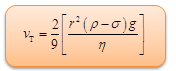Surface tension is the name of a property of liquid while force of surface tension is different from it.
For a solid-liquid pair having acute (<90º) angle of contact,

(a) the liquid wets the solids.

(b) shape of meniscus is concave upwards.

(c) liquid rises up into a capillary tube made of that solid.

For a solid-liquid pair having obtuse (>90º) angle of contact,

(a) the liquid does not wet the solids.

(b) shape of meniscus is convex upwards.

(c) the liquid is depressed in a capillary tube made of that solid.

Problem 5 (JEE Main):-

The radii of the two columns is U-tube are r1 and r2. When a liquid of density ρ (angle of contact is 0º) is filled in it, the level difference of liquid in two arms is h. Find out the surface tension of liquid.

Solution:-

We know that, h = 2T/rρg

So,  h1 = 2T/r1ρg

h2 = 2T/r2ρg

h1- h2 = h = 2T/ρg(1/r1 – 1/r2)

Thus, T = hρgr1r2/2(r2 - r1)

Problem 6 (JEE Main):-

A metal ball A (density 3.2 g/cc) is dropped in water, while another metal ball B (density 6.0 g/cc) is dropped in a liquid of density 1.6 g/cc. If both the balls have the same diameter and attain the same terminal velocity, find out the ratio of viscosity of water to that of the liquid.

Solution:-

Terminal velocity is given by,

vT = 2/9 [r2(ρ1 - ρ2)/η]g

(vT)A =  2/9 [r2(32 - 1)/ηw]g

(vT)B =  2/9 [r2(6.0 - 1.6)/ηl]g

Given, (vT)A = (vT)B

Equating these two we get, ηw/ηl = 0.5Question 1 :-

Two molecules are separated an appreciable distance apart. What is the nature of the force between them:

(a) attractive                                       (b) repulsive

(c) both attractive and repulsive         (d) none of them

Question 2 :-

The force of surface tension acts in such a direction that the curvature of the surface should:

(a) increase                (b) decrease

(c) remain the same    (d) none of these

Question 3 :-

When the temperature is increased, the angle of contact of a liquid:

(a) first increases and then decreases                 (b) decreases

(c) remains the same                                            (d) increases

Question 4:-

When a capillary tube is dipped in a liquid, the level of the liquid inside the tube rises because of:

(a) viscosity                (b) surface tension

(c) osmosis                 (d) diffusionQ.1
Q.2
Q.3
Q.4

a

a

d

b

Related Resources

You might like to refer Solved Examples on Fluid Mechanics.

For getting an idea of the type of questions asked, refer the  Previous Year Question Papers.### Course Features

• 101 Video Lectures
• Revision Notes
• Previous Year Papers
• Mind Map
• Study Planner
• NCERT Solutions
• Discussion Forum
• Test paper with Video Solution